# Work Done by Expanding Gas

The work done, w , by a gas expanding from 2 cm 3 to 10 cm 3 is given by the following definite integral: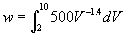.

1. Use Simpson's Rule with N = 4 subintervals to estimate w .
2. Find an exact value for w by evaluating the definite integral above using calculus.

## Solution:

1. From Simpson's Rule with N = 4 subintervals: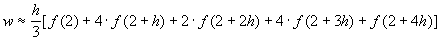where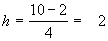, and f(V) = 500V -1.4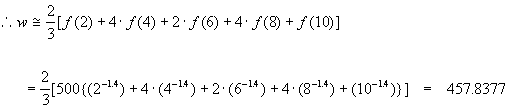2. Evaluate the definite integral using calculus: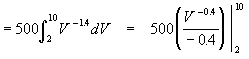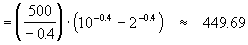Written by Arch McFarlane, June 2003